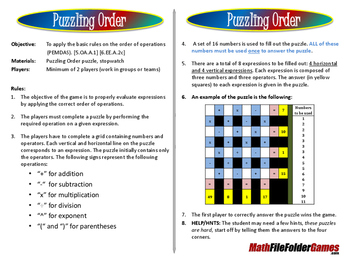# Puzzling Order an Order of Operations Game/Puzzle

“Excellent resource”Robin K.Objective: To apply the basic rules on the order of operations (PEMDAS). [5.OA.A.1] [6.EE.A.2c]
Materials: Puzzling Order puzzle
Players: Minimum of 2 players (work in groups or teams)

Rules:
The objective of the game is to properly evaluate expressions by applying the correct order of operations.

The players must complete a puzzle by performing the required operation on a given expression.

The players have to complete a grid containing numbers and operators. Each vertical and horizontal line on the puzzle corresponds to an expression. The puzzle initially contains only the operators. The following signs represent the following operations:
“-“ for subtraction
“x” for multiplication
“÷” for division
“^” for exponent
“(“ and “)” for parentheses

A set of 16 numbers is used to fill out the puzzle. ALL of these numbers must be used once to answer the puzzle.

There are a total of 8 expressions to be filled out: 4 horizontal and 4 vertical expressions. Each expression is composed of three numbers and three operators. The answer (in yellow squares) to each expression is given in the puzzle.

An example of the puzzle is the following:

HELP/HINTS: The student may need a few hints, these puzzles are hard, start off by telling them the answers to the four corners.This Game/Puzzle is included in my 6th Grade File Folder Math Games Printable Book